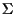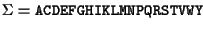Next: Definitions Up: Probabilistic ancestral sequences and Previous: Probabilistic ancestral sequences and

# Introduction

In our view the main contribution of this paper is to introduce the computation of probabilities of a given evolutionary configuration (EC), that is a phylogenetic tree with sequences at its leaves, based on a Markovian model of evolution. From this probabilities, we can compute the probability profile at any internal node or internal branch of the phylogenetic tree, and when these are computed at the root of the tree, we call them probabilistic ancestral sequences (PAS). The ability to compute probabilities of particular configurations, allows us to use a dynamic programming (DP) algorithm to align two subtrees. By starting from the leaves and working towards the root of the tree with this procedure, we construct a multiple alignment of all the sequences.

The problem of computing multiple alignments is a difficult one. All of the existing algorithms [7,2,5,11,14,19,16,12,1,13] are either heuristic, or require exponential time. The algorithm here described is the first one to work in polynomial time and to be founded on an accepted model of evolution. These methods can be applied to DNA, RNA, natural language or any other similar problem. We will denote bythe alphabet over which these sequences are built. All of our algorithms work for arbitrary alphabets. In this paper, all the examples are on proteins. This particular case can be summarized as being over an alphabet of 20 symbols, the letters.

This paper will not discuss how to construct the phylogenetic trees, this subject falls beyond our present scope. The algorithms we describe assume we start with the sequences and a phylogenetic tree.Next: Definitions Up: Probabilistic ancestral sequences and Previous: Probabilistic ancestral sequences and
Gaston Gonnet
1998-07-14